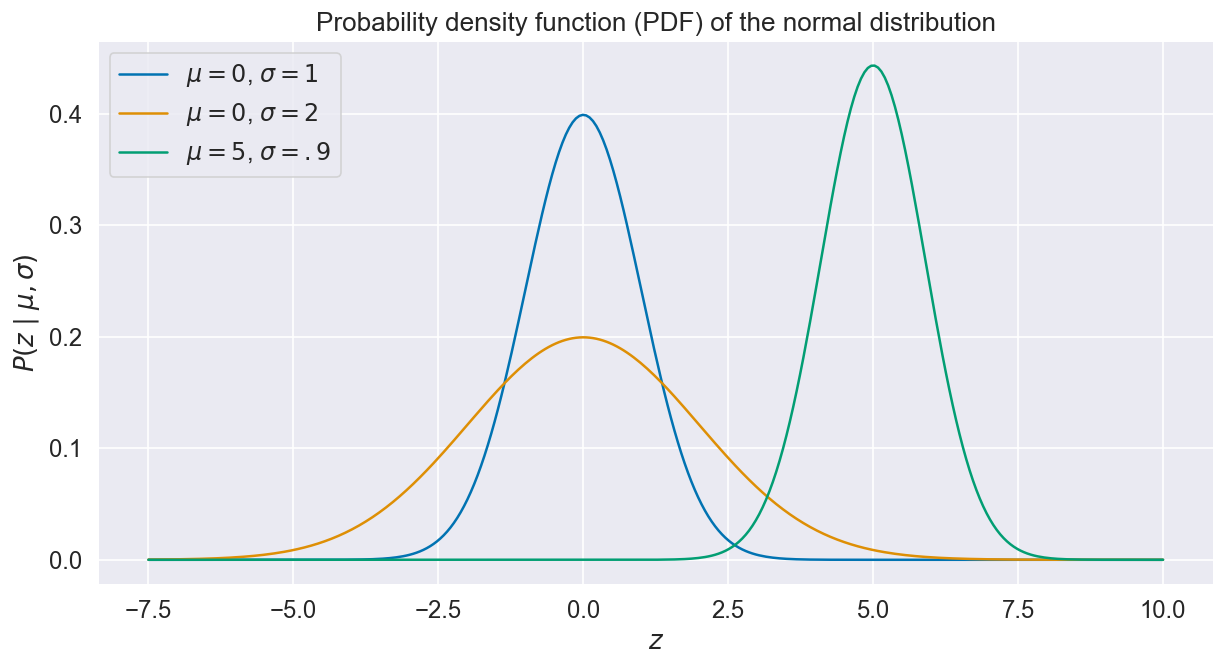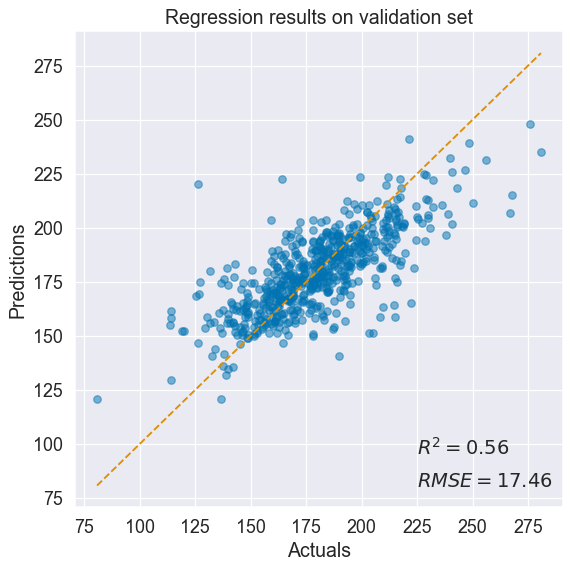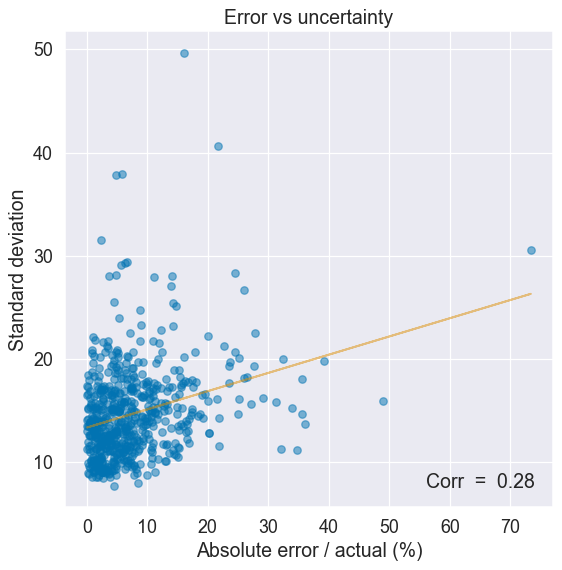Understanding and modeling uncertainty surrounding a machine learning prediction is of critical importance to any “real life” project. It provides a handle to deal with cases where the model strays too far away from its domain of applicability, into territories where using the prediction would be inacurate or downright dangerous. Think medical diagnosis or self-driving cars.

It is often useful to divide uncertainty into two distinct quantities.

The first kind, which we refer to as aleatoric uncertainty, is concerned with uncompressible errors which have to do, e.g., with measurement, or with the underlying physical limitations of the system we are modeling. A differentiator of aleatoric uncertainty is that more data won’t help reduce it.

The second kind, dubbed epistemic uncertainty, i.e. relating to knowledge, is a measure of surprise. Imagine a self-driving car exposed to a hail storm for the first time. Modeled properly, epistemic uncertainty ought to be high in this situation, possibly triggering an emergency pull over. In this case, training the model on more data would help reduce uncertainty.

In this series, we’ll look at how to model such uncertainty using plain PyTorch, via the distributions module. This first part of the series is concerned with modeling aleatoric uncertainty, while part 2 will be looking into epistemic uncertainty.

## Modeling Aleatoric Uncertainty

The problem can be framed as follows: given a dataset of inputs $x$, of dimension $d$, and their respective noisy outputs $y$ of dimension $m$, the goal is to find a function $f : \mathbb{R}^d \mapsto \mathbb{R}^m$ such as:

$f(x) = \mu(x) + \epsilon(x)$

Where $\mu(x) = \mathbb{E}[f(x)]$ is the expectation (i.e. mean) of $f$ and $\epsilon$ represents the uncompressible error (i.e. aleatoric uncertainty) around that expected value.

We’ll start with a straightforward linear regression example where $d = m = 1$ and $\mu(x)$ is a linear combination of x, before moving on to a more interesting neural network example.

### Example 1 — Linear RegressionThe data looks fairly linearly distributed, with a little bit of random noise around the outputs (which is exactly how this toy dataset was generated). Therefore, we’ll tentatively define our function $f$ as a linear combination of $x$ plus some noise:

$f(x) = \alpha x + \beta + \epsilon$

Where $\alpha$ is the slope, $\beta$ is the intercept and $\epsilon$ is the aleatoric uncertainty, which we’ll assume is independent of $x$ in this example.

As the name suggests, the aleatoric uncertainty term $\epsilon$ is random by nature, and therefore is best modeled by a probability distribution. The noise looks symmetrical around $y$, so a good option is to model uncertainty with the well known normal distribution $\mathcal{N}$, which produces values equal to the distribution mean $\mu$ plus or minus a few standard deviations $\sigma$:

$\tag{i} z \sim \mathcal{N}(\mu, \sigma) \iff P(z \mid \mu, \sigma) = \frac{1}{\sigma\sqrt{2\pi}} exp\{-\frac{1}{2}(\frac{z - \mu}{\sigma})^2\}$

Here, “$\sim$” merely means “sampled from”, and $P(z \mid \mu, \sigma)$ is the probability density function (PDF), a quantity that determines the likelihood of a value $z$ given the distribution’s mean and standard deviation. Examples of Normal PDFs are shown below for various values of $\mu$ and $\sigma$.Coming back to our example, and now equipped with this new formalism, let’s redefined $f(x)$ as a normally distributed variable with mean $\alpha x + \beta$ and standard deviation $\sigma$:

$f(x) \sim \mathcal{N}(\alpha x + \beta, \sigma)$

In plain english, this expression tells us that the function $f(x)$ takes on the value $\alpha x + \beta$ on average, plus or minus some error $\sigma$, i.e. exactly what we are looking for.

What’s left is to find appropriate values for our three parameters $\alpha$, $\beta$ and $\sigma$.

As per equation (i), we know that the likelihood of observing a value $y$ given our model parameters is:

$\tag{ii} P(y \mid x, \alpha, \beta, \sigma) = \frac{1}{\sigma\sqrt{2\pi}} exp\{-\frac{1}{2}(\frac{y - (\alpha x + \beta)}{\sigma})^2\}$

Therefore, we’d like to find the values of $\alpha$, $\beta$ and $\sigma$ that maximize the quantity $P(y \mid x, \alpha, \beta, \sigma)$, i.e. that maximize the likelihood of observing $y$ given the input $x$ plus our model assumptions.

This procedure is appropriately called Maximum Likelihood Estimation (MLE). We’ll be using the Python library PyTorch to optimize (ii) with regard to our model parameters.

First, we start by defining a PyTorch model:

• Parameters are randomly initialized in the __init__ function.
• In forward, we explictly return an instance of Normal, parametrized by $\mu = \alpha x + \beta$ and $\sigma$.
• The standard deviation $\sigma$ must be positive, which is why we are mapping the underlying parameter $s$ from $\mathbb{R}$ to $\mathbb{R}^{+}$ via the softplus function, shown below:Next, we define the training procedure:

There isn’t much to say here as this function is pretty much boilerplate. The interesting part is the compute_loss function, defined below:

A few things worth noting:

• As per equation (ii), we are looking to maximize the likelihood of observing the output values $y$.
• However, PyTorch expects to be minimizing a function, thus why we are negating the quantity.
• Moreover, the exponential term of equation (ii) does not play well with numerical optimization: values can get arbitrary large, causing overflow. That’s why it is customary to take the natural logarithm of the likelihood function. Distribution objects provide a log_prob method.
• Finally, the likelihoods of all the outputs $y$ are combined in a single value. In probability theory, combining independent events is done by taking the product of individual probabilities. Since we took the logarithm of these probabilities, we simply need to sum up the values.
• This loss function is often referred to as the negative log-likelihood.

After running train_one_step several times, we get the following result:Hooray! The training procedure did a good job fitting the model parameters $\alpha$, $\beta$ and $\sigma$. For further details and complete code, see the Linear Regression Jupyter Notebook.

### Example 2 — Feed Forward Neural Network

Let’s now turn to a more realistic example. We’ll use data from the OLS Regression Challenge, where the goal is to predict cancer mortality rates in US counties based on a number of socio-demographic variables such as median age, income, poverty rate, unemployment rate, etc.

Once again, we’ll model the aleatoric uncertainty surrounding the predictions with a normal distribution. This time though, instead of assuming that $\mu$ is a linear combination of $x$ and that $\sigma$ is a single value, independent of $x$, we’ll model both parameters with a feed forward neural network.

Formally, our function $f$ takes the following form:

$f(x) \sim \mathcal{N}(\mu(x, \Theta), \sigma(x, \Theta))$

Where $\Theta$ represents the parameters of the neural network used to model $\mu$ and $\sigma$.

let’s get on to the implementation:

The mean $\mu(x, \Theta)$ and standard deviation $\sigma(x, \Theta)$ share an internal representation of $x$ via the first layer of the network, before branching out to build their own representations. Once again, the standard deviation parameter is passed through the softplus function to ensure positivity.

All that is left is to train the network. Remarkably, the loss function and training step defined for the linear regression example can be used with no change: the API in both cases is the same, as the model accepts an input $x$ and returns a Normal distribution object.

Goodness of fit after training is shown in the figure below:The model does a decent job at predicting cancer mortality rates.

How good is our estimate of aleatoric uncertainty? To find out, let’s plot uncertainty as a function of the absolute error in our prediction. A higher uncertainty should correlate with higher average error and vice versa.Indeed, uncertainty seems to grow proportionally to the absolute error in our predictions!

For further details and complete code, see the Neural Network Jupyter Notebook.

### Take Away

PyTorch’s distributions module provides an elegant way to parametrize probability distributions.

In this post, we looked at modeling uncertainty using the Normal distribution, but there are a plethora of other distributions available for different problems (see for example the Categorical distribution, handy for classification tasks).

Most of these distributions provide an implementation of the log-likelihood function via the log_prob method, which can be used directly when defining the loss function.

In part 1, we’ve been focusing on the aleatoric uncertainty, i.e. the uncompressible error inherent to every dataset (e.g. because of measurement error, or a lack of predictive features, etc.)

In part 2, we’ll be looking at epistemic uncertainty, or the measure of surprise. Stay tuned!